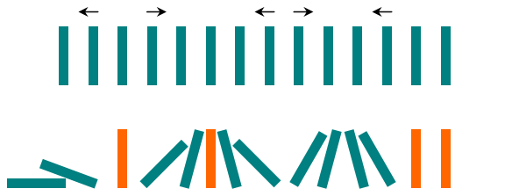Formatted question description: https://leetcode.ca/all/838.html

# 838. Push Dominoes

Medium

## Description

There are N dominoes in a line, and we place each domino vertically upright.

In the beginning, we simultaneously push some of the dominoes either to the left or to the right.After each second, each domino that is falling to the left pushes the adjacent domino on the left.

Similarly, the dominoes falling to the right push their adjacent dominoes standing on the right.

When a vertical domino has dominoes falling on it from both sides, it stays still due to the balance of the forces.

For the purposes of this question, we will consider that a falling domino expends no additional force to a falling or already fallen domino.

Given a string “S” representing the initial state. S[i] = 'L', if the i-th domino has been pushed to the left; S[i] = 'R', if the i-th domino has been pushed to the right; S[i] = '.', if the i-th domino has not been pushed.

Return a string representing the final state.

Example 1:

Input: “.L.R…LR..L..”

Output: “LL.RR.LLRRLL..”

Example 2:

Input: “RR.L”

Output: “RR.L”

Explanation: The first domino expends no additional force on the second domino.

Note:

1. 0 <= N <= 10^5
2. String dominoes contains only 'L', 'R' and '.'

## Solution

First, collect all the indices of character 'L' and character 'R', using negative and positive indices respectively.

Next, deal with dominoes with the collected indices.

Among the collected indices, if the leftmost index is negative, then the dominoes left to the index all become 'L'. If the rightmost index is positive, then the dominoes right to the index all become 'R'.

For the remaining collected indices, consider each adjacent indices pair. If the left index is zero or positive and the right index is negative, then the dominoes between the two indices are pushed in two directions, and the middle index should be considered. Otherwise, the dominoes between the two indices are pushed in the same direction.

class Solution {
public String pushDominoes(String dominoes) {
char[] array = dominoes.toCharArray();
int length = array.length;
List<Integer> indices = new ArrayList<Integer>();
for (int i = 0; i < length; i++) {
char c = array[i];
if (c == 'L')
else if (c == 'R')
}
int size = indices.size();
if (size == 0)
return dominoes;
int firstIndex = indices.get(0), lastIndex = indices.get(size - 1);
if (firstIndex < 0) {
for (int i = -firstIndex - 1; i >= 0; i--)
array[i] = 'L';
}
if (lastIndex >= 0) {
if (lastIndex > 0 || array == 'R') {
for (int i = lastIndex + 1; i < length; i++)
array[i] = 'R';
}
}
for (int i = 1; i < size; i++) {
int index1 = indices.get(i - 1), index2 = indices.get(i);
if (index1 >= 0 && array[index1] == 'R' && index2 < 0) {
index2 = Math.abs(index2);
if (index1 % 2 == index2 % 2) {
int mid = (index2 - index1) / 2 + index1;
for (int j = index1 + 1; j < mid; j++)
array[j] = 'R';
for (int j = index2 - 1; j > mid; j--)
array[j] = 'L';
} else {
int midLeft = (index2 - index1) / 2 + index1;
for (int j = index1 + 1; j <= midLeft; j++)
array[j] = 'R';
for (int j = index2 - 1; j > midLeft; j--)
array[j] = 'L';
}
} else if (index1 >= 0 && array[index1] == 'R') {
for (int j = index1 + 1; j < index2; j++)
array[j] = 'R';
} else if (index2 < 0) {
for (int j = -index1 + 1; j < -index2; j++)
array[j] = 'L';
}
}
return new String(array);
}
}